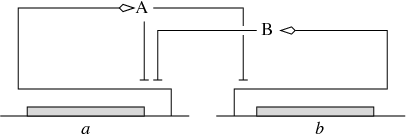EN FR
EN FR

## Section: Research Program

### Analysis of qualitative dynamics of gene regulatory networks

Participants : Hidde de Jong [Correspondent] , Michel Page.

The dynamics of gene regulatory networks can be modeled by means of ordinary differential equations (ODEs), describing the rate of synthesis and degradation of the gene products as well as regulatory interactions between gene products and metabolites. In practice, such models are not easy to construct though, as the parameters are often only constrained to within a range spanning several orders of magnitude for most systems of biological interest. Moreover, the models usually consist of a large number of variables, are strongly nonlinear, and include different time-scales, which makes them difficult to handle both mathematically and computationally. This has motivated the interest in qualitative models which, from incomplete knowledge of the system, are able to provide a coarse-grained picture of its dynamics.

A variety of qualitative modeling formalisms have been introduced over the past decades. Boolean or logical models, which describe gene regulatory and signalling networks as discrete-time finite-state transition systems, are probably most widely used. The dynamics of these systems are governed by logical functions representing the regulatory interactions between the genes and other components of the system. IBIS has focused on a related, hybrid formalism that embeds the logical functions describing regulatory interactions into an ODE formalism, giving rise to so-called piecewise-linear differential equations (PLDEs, Figure 2). The use of logical functions allows the qualitative dynamics of the PLDE models to be analyzed, even in high-dimensional systems. In particular, the qualitative dynamics can be represented by means of a so-called state transition graph, where the states correspond to (hyperrectangular) regions in the state space and transitions between states arise from solutions entering one region from another.

First proposed by Leon Glass and Stuart Kauffman in the early seventies, the mathematical analysis of PLDE models has been the subject of active research for more than four decades. IBIS has made contributions on the mathematical level, in collaboration with the BIOCORE and BIPOP project-teams, notably for solving problems induced by discontinuities in the dynamics of the system at the boundaries between regions, where the logical functions may abruptly switch from one discrete value to another, corresponding to the (in)activation of a gene. In addition, many efforts have gone into the development of the computer tool Genetic Network Analyzer (GNA) and its applications to the analysis of the qualitative dynamics of a variety of regulatory networks in microorganisms. Some of the methodological work underlying GNA , notably the development of analysis tools based on temporal logics and model checking, which was carried out with the Inria project-teams CONVEX (ex-VASY) and POP-ART, has implications beyond PLDE models as they apply to logical and other qualitative models as well.

Figure 2. (Left) Example of a gene regulatory network of two genes (a and b), each coding for a regulatory protein (A and B). Protein B inhibits the expression of gene a, while protein A inhibits the expression of gene b and its own gene. (Right) PLDE model corresponding to the network in (a). Protein A is synthesized at a rate ${\kappa }_{a}$, if and only if the concentration of protein A is below its threshold ${\theta }_{a}^{2}$ (${x}_{a}<{\theta }_{a}^{2}$) and the concentration of protein B below its threshold ${\theta }_{b}$ (${x}_{b}<{\theta }_{b}$). The degradation of protein A occurs at a rate proportional to the concentration of the protein itself (${\gamma }_{a}\phantom{\rule{0.166667em}{0ex}}{x}_{a}$).$\begin{array}{cc}& {\stackrel{˙}{x}}_{a}={\kappa }_{a}\phantom{\rule{0.166667em}{0ex}}{s}^{-}\left({x}_{a},{\theta }_{a}^{2}\right)\phantom{\rule{0.166667em}{0ex}}{s}^{-}\left({x}_{b},{\theta }_{b}\right)-{\gamma }_{a}\phantom{\rule{0.166667em}{0ex}}{x}_{a}\hfill \\ & {\stackrel{˙}{x}}_{b}={\kappa }_{b}\phantom{\rule{0.166667em}{0ex}}{s}^{-}\left({x}_{a},{\theta }_{a}^{1}\right)-{\gamma }_{b}\phantom{\rule{0.166667em}{0ex}}{x}_{b}\hfill \\ & {s}^{+}\left(x,\theta \right)=\left\{\begin{array}{cc}1,\hfill & \phantom{\rule{4.pt}{0ex}}\text{if}\phantom{\rule{4.pt}{0ex}}x>\theta \hfill \\ 0,\hfill & \phantom{\rule{4.pt}{0ex}}\text{if}\phantom{\rule{4.pt}{0ex}}x<\theta \hfill \end{array}\right\\hfill \\ & {s}^{-}\left(x,\theta \right)=1-{s}^{+}\left(x,\theta \right)\hfill \end{array}$